# Tuning for force tracking in MR (Magneto-Rheological) dampers

Shuhaibul
I already create MR damper modelling by using non-parametric linearised data driven (NPLDD) double input model. The hysteresis curve behavior is 99.9% followed as per experimental data, Figure below: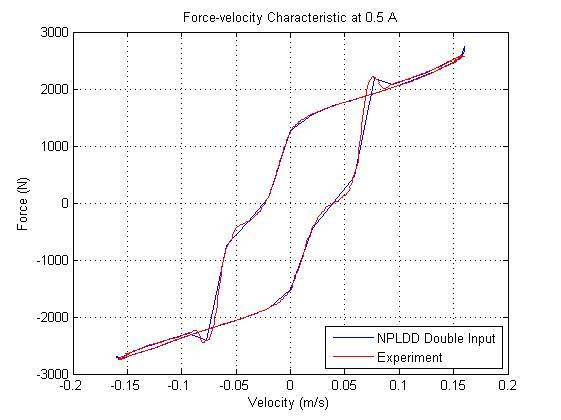Then, by using this model, I had created closed loop model for force tracking control. The closed loop is developed by using continuous state control and If-Then Rules block as below: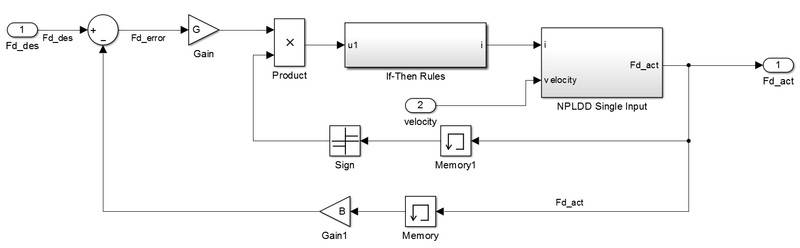The input Fd_des and velocity is set to be sine, square, and sawtooth waveform, so that the output (Fd_act) will exhibit the same as input (Fd_des). However, after several time tuning the value of G and B, the result for force tracking (F_act vs F_des) is not the same as below: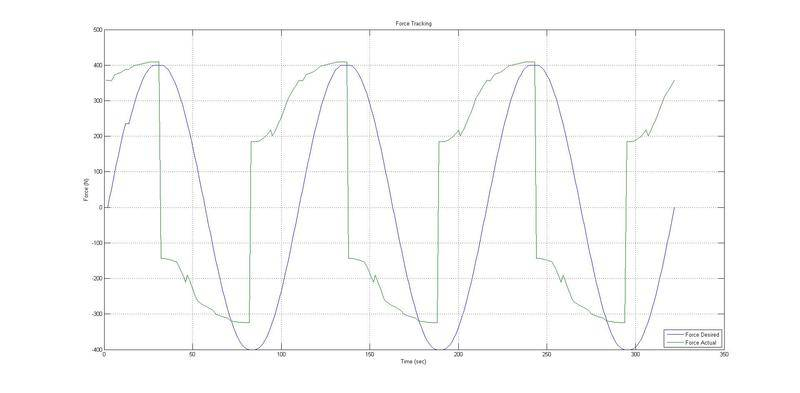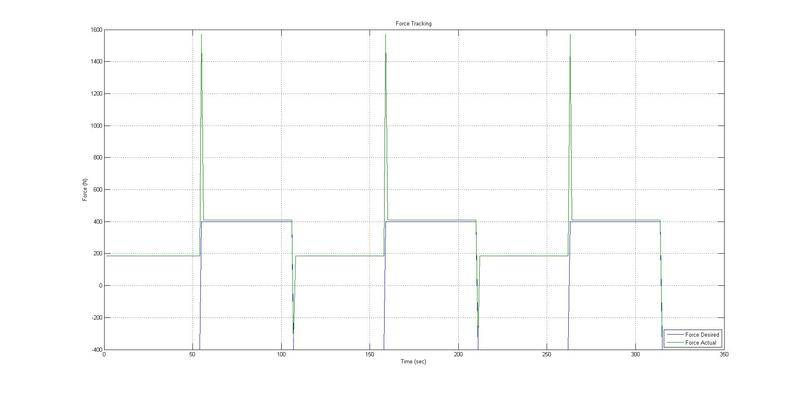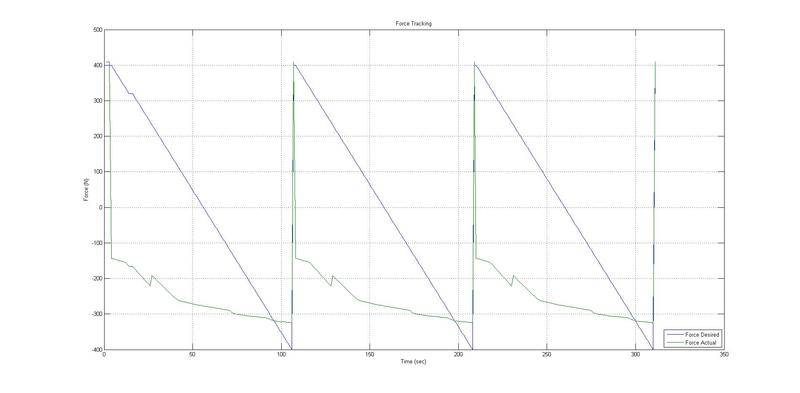All the result is not followed/tracked the input. The value of G and B are 0.05 and 2 respectively.